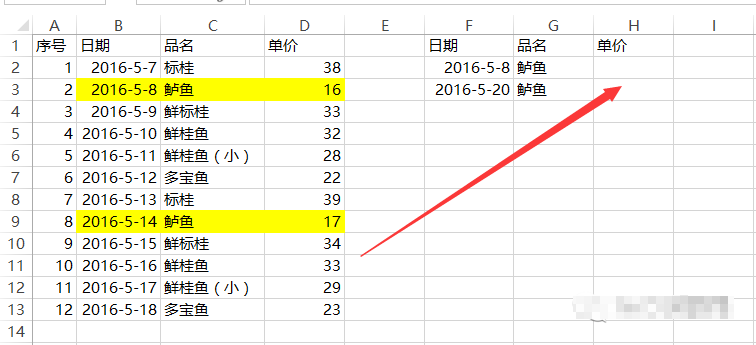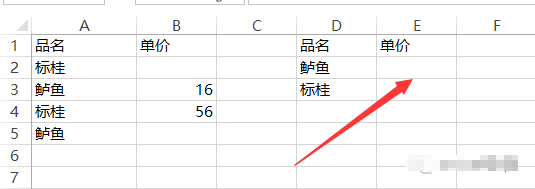# 关于Excel查找对应值的几个常见问题探讨及解决方法=VLOOKUP(F2,A:D,4,0)

=VLOOKUP(F2,C:D,2,0)=LOOKUP(1,0/((\$C\$2:\$C\$13=G2)*(\$B\$2:\$B\$13<=F2)),\$D\$2:\$D\$13)=LOOKUP(1,0/((\$A\$2:\$A\$5=D2)*(\$B\$2:\$B\$5<>"")),\$B\$2:\$B\$5)

=SUMIF(A:A,D2,B:B)

tag : 查找